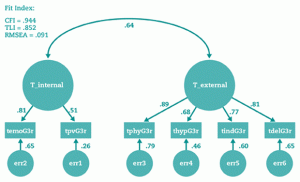# Exploratory factor analysis vs confirmatory factor analysisThis article will discuss differences between exploratory factor analysis and confirmatory factor analysis. Exploratory factor analysis is abbreviated wit EFA , while the confirmatory factor analysis known as CFA .

## About Exploratory Factor Analysis (EFA)

EFA is a statistical method to build structural model consisting set of variables. EFA is one of the factor analysis method to identify the relationship between the manifest variables in building a construct. Researcher also mention manifest variables as indicators variable. A researcher uses EFA when he does not have a beginning information in grouping set of indicators. So researchers set of indicators (manifest) then create variables. In conditions where the latent variables does not have clear indicators, the EFA is an appropriate method. Possibly, indicators of the latent variable indicators of possible overlap with other latent variables.

Researchers can use SPSS software to analyze EFA. All data of indicator input into the software. Therefore there is no assumption group of indicators. In EFA, we do not know how many factors or latent variables will create. Although researchers allow to determine how many the expected number of factors.

Factor loading is a measurement indicating into which group an indicator will gather. When the value is greater then these, then indicators will gather in the same factors.

## About Confirmatory Factor Analysis (CFA)

CFA is one of factor analysis, commonly in social research. This method examines whether statistically the indicators gather consistently in a group. In the CFA, researchers test whether the data fit to the model established previously or not. The fundamental difference between the CFA and EFA is: in the CFA, researchers have prior assumption that indicators fit into a certain latent variables. Researcher has develop a hypothetical model based on the theoretical framework or previous studies referenced.

Therefore, there is an established model to examine, then the CFA test the model. CFA is a part of Structural Equation Modeling (SEM).

Fitness Measurement in CFA is the same with SEM fitness index. Chi Square, RMSEA, GFI, AGFI are some fitness index to use beyond the weighted value of each indicator.

The similarity of EFA and CFA

One of the similarity between EFA and CFA is a variance to measure the contribution of construct variables.